# Seven Segment Displays Digital Electronics Seven Segment Displays

• Slides: 16Seven Segment Displays Digital Electronics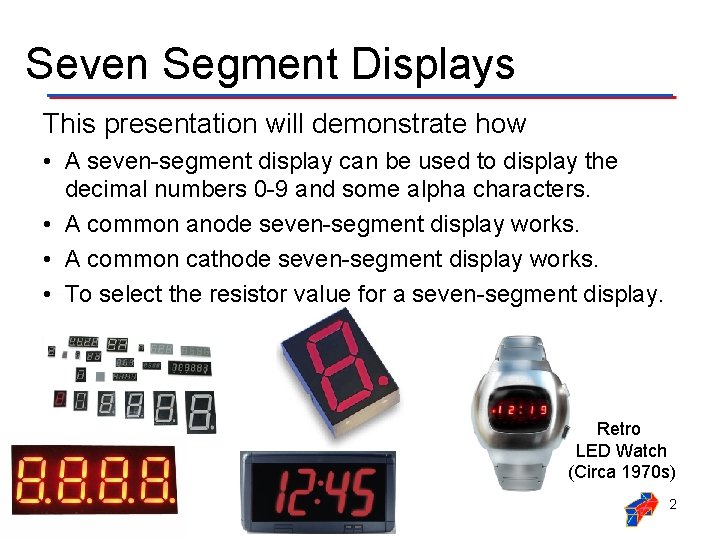Seven Segment Displays This presentation will demonstrate how • A seven-segment display can be used to display the decimal numbers 0 -9 and some alpha characters. • A common anode seven-segment display works. • A common cathode seven-segment display works. • To select the resistor value for a seven-segment display. Retro LED Watch (Circa 1970 s) 2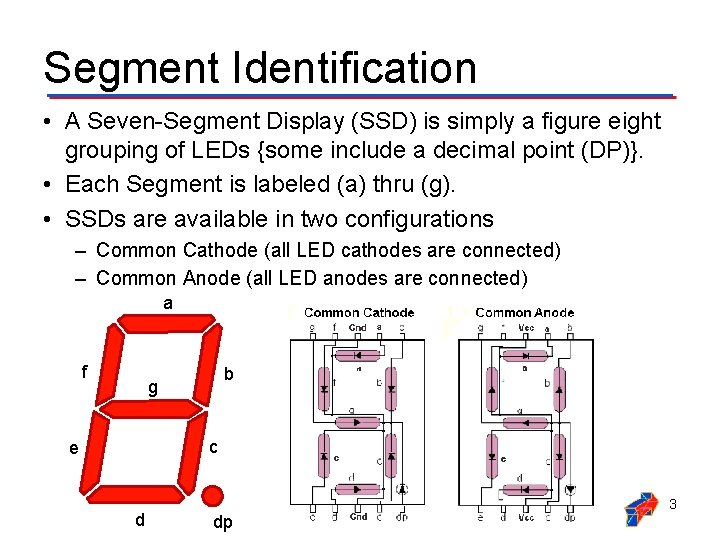Segment Identification • A Seven-Segment Display (SSD) is simply a figure eight grouping of LEDs {some include a decimal point (DP)}. • Each Segment is labeled (a) thru (g). • SSDs are available in two configurations – Common Cathode (all LED cathodes are connected) – Common Anode (all LED anodes are connected) a f b g c e d dp 3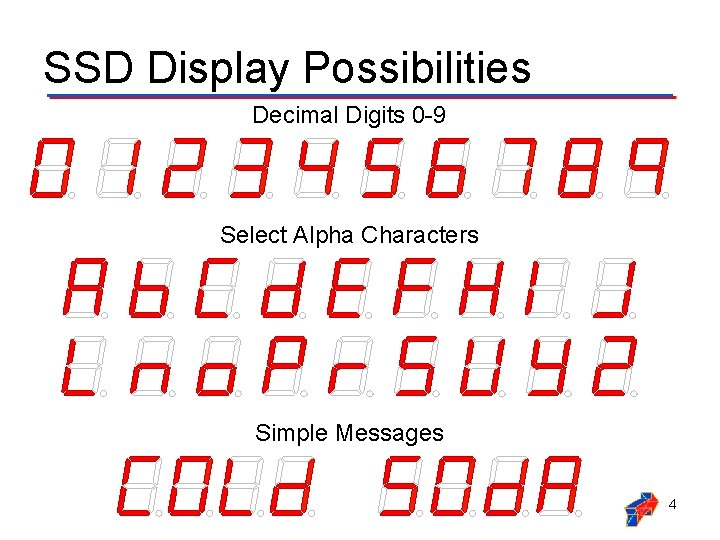SSD Display Possibilities Decimal Digits 0 -9 Select Alpha Characters Simple Messages 4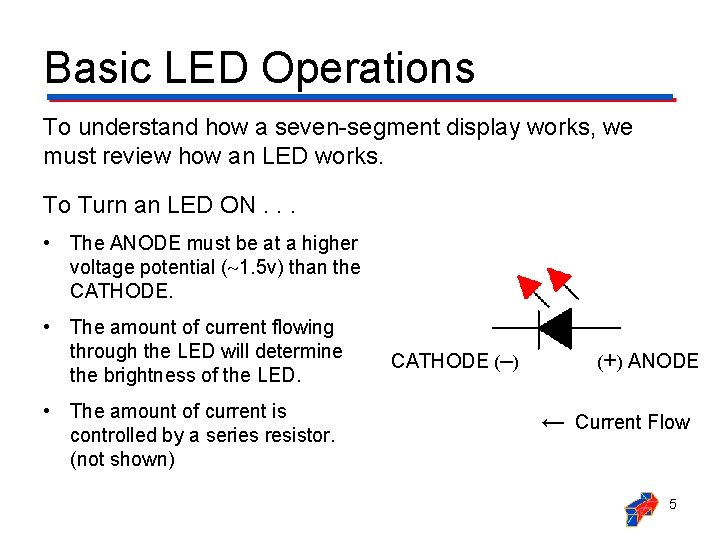Basic LED Operations To understand how a seven-segment display works, we must review how an LED works. To Turn an LED ON. . . • The ANODE must be at a higher voltage potential ( 1. 5 v) than the CATHODE. • The amount of current flowing through the LED will determine the brightness of the LED. • The amount of current is controlled by a series resistor. (not shown) CATHODE (‒) (+) ANODE ← Current Flow 5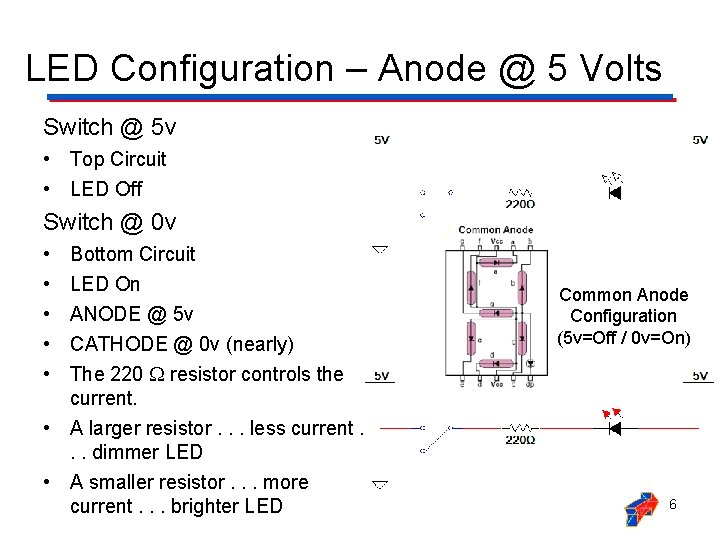LED Configuration – Anode @ 5 Volts Switch @ 5 v • Top Circuit • LED Off Switch @ 0 v • • • Bottom Circuit LED On ANODE @ 5 v CATHODE @ 0 v (nearly) The 220 resistor controls the current. • A larger resistor. . . less current. . . dimmer LED • A smaller resistor. . . more current. . . brighter LED Common Anode Configuration (5 v=Off / 0 v=On) 6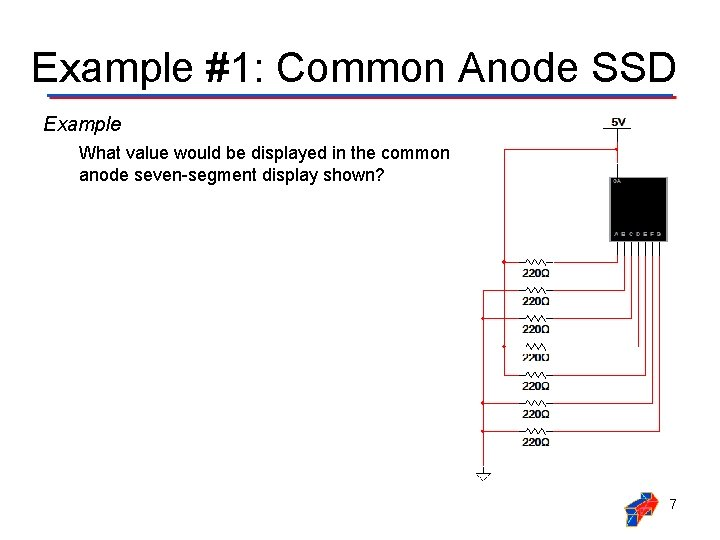Example #1: Common Anode SSD Example What value would be displayed in the common anode seven-segment display shown? 7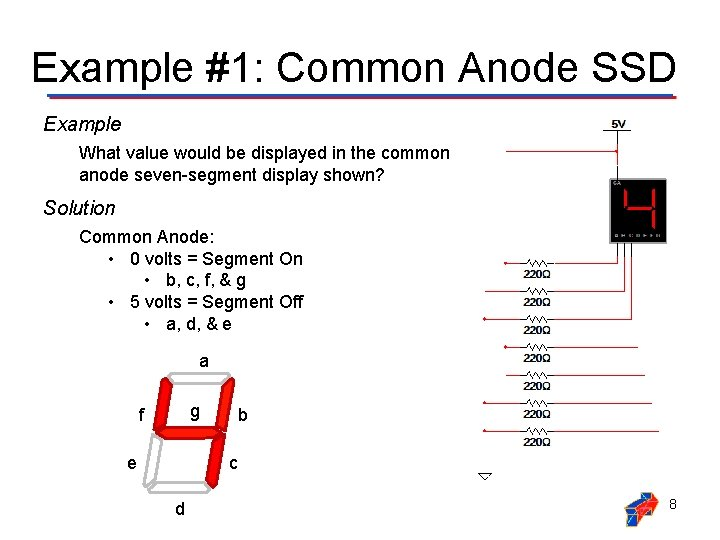Example #1: Common Anode SSD Example What value would be displayed in the common anode seven-segment display shown? Solution Common Anode: • 0 volts = Segment On • b, c, f, & g • 5 volts = Segment Off • a, d, & e a g f b c e d 8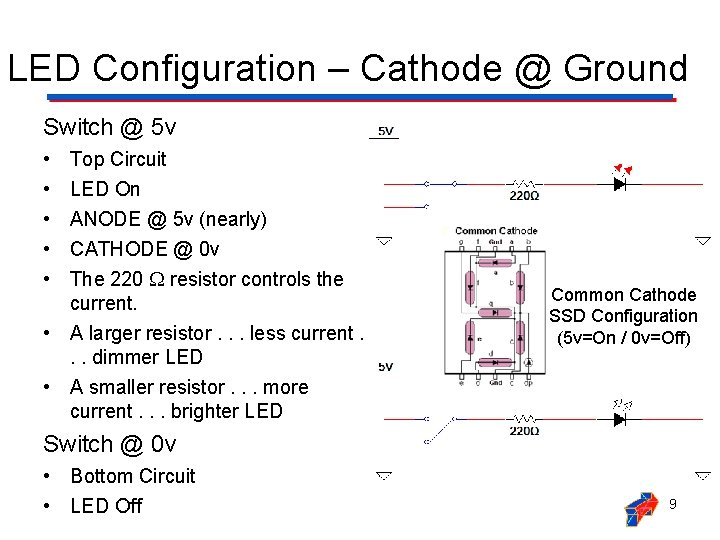LED Configuration – Cathode @ Ground Switch @ 5 v • • • Top Circuit LED On ANODE @ 5 v (nearly) CATHODE @ 0 v The 220 resistor controls the current. • A larger resistor. . . less current. . . dimmer LED • A smaller resistor. . . more current. . . brighter LED Common Cathode SSD Configuration (5 v=On / 0 v=Off) Switch @ 0 v • Bottom Circuit • LED Off 9Example #2: Common Cathode SSD Example What value would be displayed in the common cathode seven-segment display shown? 10Example #2: Common Cathode SSD Example What value would be displayed in the common cathode seven-segment display shown? Solution Common Cathode: • 5 volts = Segment On • a, b, d, e, & g • 0 volts = Segment Off • c&f a g f b c e d 11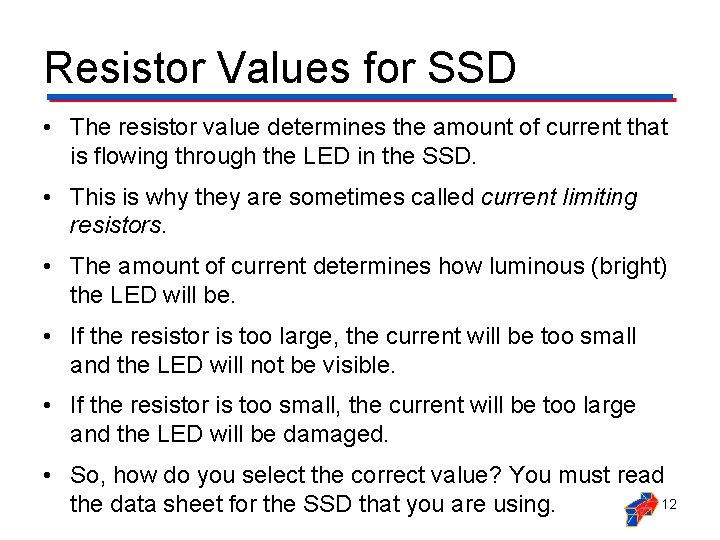Resistor Values for SSD • The resistor value determines the amount of current that is flowing through the LED in the SSD. • This is why they are sometimes called current limiting resistors. • The amount of current determines how luminous (bright) the LED will be. • If the resistor is too large, the current will be too small and the LED will not be visible. • If the resistor is too small, the current will be too large and the LED will be damaged. • So, how do you select the correct value? You must read 12 the data sheet for the SSD that you are using.A Review of Circuit Theory • The diagram below is a single segment of a common anode seven-segment display. • The voltage across the LED (when on) is 1. 5 volts. • Using Kirchhoff's Voltage Law, we know that the voltage across the resistor is 3. 5 volts (i. e. , 5 v – 1. 5 v = 3. 5 v). • Thus, using Ohm’s Law, we can calculate the value of the resistor if we know the current that is to flow through the LED. ← I 13Selecting A Resistor Value LTS-4801 JR Common Anode Seven-Segment Display Luminous Intensity vs. Forward Current Graph • Let’s arbitrarily pick a luminous intensity of 1. 5 (not too bright, not too dim). • From the graph, we need a current of 15 m. A. • Using Ohm’s Law : 14Example #3: Resistor Value Example Calculate the resistor value required to have a luminous intensity of 2. 5. 15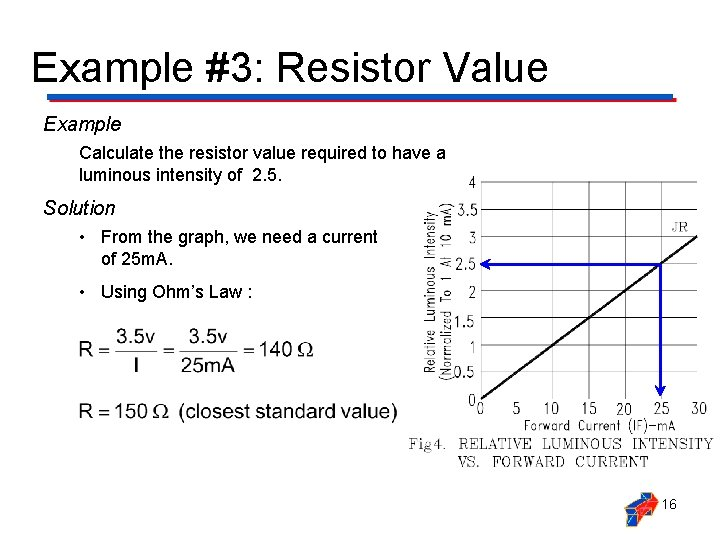Example #3: Resistor Value Example Calculate the resistor value required to have a luminous intensity of 2. 5. Solution • From the graph, we need a current of 25 m. A. • Using Ohm’s Law : 16